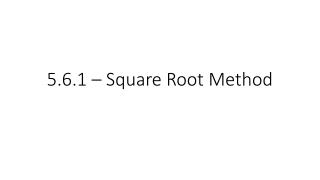DownloadDownload Presentation5.6.1 – Square Root Method

# 5.6.1 – Square Root Method

Download Presentation## 5.6.1 – Square Root Method

- - - - - - - - - - - - - - - - - - - - - - - - - - - E N D - - - - - - - - - - - - - - - - - - - - - - - - - - -
##### Presentation Transcript

1. 5.6.1 – Square Root Method

2. Recall, we solved “quadratic equations” when we set a polynomial equation equal to 0 • Example. x2 + 5x + 6 = 0

3. In some cases, we can use a special method to solve the equations • So far we have used factoring, calculators, quadratic equation

4. Properties of Square Roots • Before we start to solve them using the new method, there are some basic properties of square roots we should know • Product Property; • Quotient Property; • In a fraction, you may not have a radical in the denominator (bottom)

5. Simplifying/Rationalizing • To simplify a radical expression, we will look for any perfect square roots we could pull out • Perfect Roots = 1, 4, 9, 16, 25, 36, 49, 64, 81, 100, 121,… • If there are no perfect roots to pull out, then the expression is considered simplified

6. Example. Simplify • Perfect root that is a factor of 18? • Example. Simplify

7. Example. Simplify • Example. Simplify

8. Rationalizing • If a fraction has a square root in the denominator, we will eliminate the radical by rationalizing • For a number a, = a • To eliminate the radical, multiply top and bottom by the radical itself • Be sure to simplify the top as necessary

9. Example. Simplify the expression • Example. Simplify the expression

10. Example. Simplify the expression • Example. Simplify the expression

11. Simplify the following expressions together. • 1) • 2) • 3) • 4)

12. Assignment • Pg. 258 • 18 – 44 even